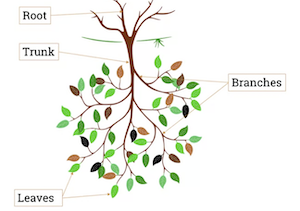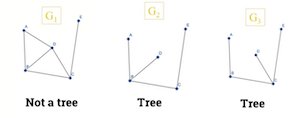## 8.101 Introduction

• Trees are usually represented upside down in Computer Science.• Trees have many use cases:

• Organisation charts.
• Computer file systems.
• Used to find shortest path in graph.
• Used to construct efficient algorithms to locate items in a list.
• Used in games such as checkers and chess to determine winning strategies.
• Use to model procedures carried out as a sequence of decisions.
• Binary tree is fundamental data structure in high-level programming.

## 8.103 Definition of a tree

• Acyclic Graph

• A graph G is called an acyclic graph if and only if G has no cycles.
• No loops and no parallel edges.
• $G_1$ contains a cycle B, C, D, E
• $G_2$ contains no cycle• Definition of a tree

• A tree is a connected acyclic undirected graph.
• Hence, a tree can have neigher loops nor edges.• A disconnected graph containing no cycles is called a forest.• Theorem 1

• An undirected graph is a tree if and only if there is a unique simple path between any 2 of its vertices.
• We can prove by contradiction
• In this example, we show that if there exists a 2nd path P2 between B and I, we can see that this results in a cycle. Hence, it is not a tree.• Theorem 2

• A tree with n vertices has n-1 edges.• Rooted trees

• A rooted tree is when one vertex has been designated as the root, and every edge is directed away from the root.

## 8.105 Spanning trees of a graph

• Spanning Trees

• In many real-life problems like Internet multicasting, we need to identify trees that exist within a graph.
• A spanning tree of graph G is a connected sub graph of G which contains all vertices of G, but with no cycles.
• Example

• $G$ is a connected graph, $T_1$, $T_2$, $T_3$ and $T_4$ are spanning trees.• To get a spanning tree of graph G.

1. Keep all verticies of G.
1. Break all cycles but keep the tree connected.
• Examples of spanning trees• Two spanning trees are said to be isomorphic if there is a bijection preserving adjaceny between the two trees.

• Some spanning trees of a graph might be isomorphic to each other: ie they're the same.* In this example, we would only draw $T_1$ and $T_2$, or $T_3$ and $T_2$ or $T_4$ and $T_2$ if we were asked for non-isomorphic trees.


## 8.107 Min Spanning Tree

• Example of a use case.
• Suppose you want to supply houses with:
• electric power
• water pipes
• sewage lines
• telephone lines
• To keep costs down, you could connect with spanning tree (power lines, for example)
• However, houses are not equal distance apart.
• To reduce costs even further, connect the houses with a minimum-cost spanning tree.
• Spanning trees costs
• Suppose you have a connected undirected graph with a weight (or cost) associated with each edge.
• The cost of a spanning tree would be the sum of the costs of its edges.
• Weight of a spanning tree

• In this image, there are 3 spanning trees of graph $G$ each with separate costs• Minimum spanning trees

• Minimum-cost spanning tree is a spanning tree that has the lowest weight (lowest cost).
• Finding spanning trees
• There are 2 algorithms for finding minimum-cost spanning trees, and both are greedy algorithms:
• Kruskal's Algorithm
• Prim's Algorithm
• Kruskal's Algorithm

• Repeatedly: keep adding cheapest edge that does not create a cycle.
• Step 1.• Step 2.• And so on...
• Prim's Algorithm